Examples

Chapter 11 Class 12 Three Dimensional Geometry
Serial order wise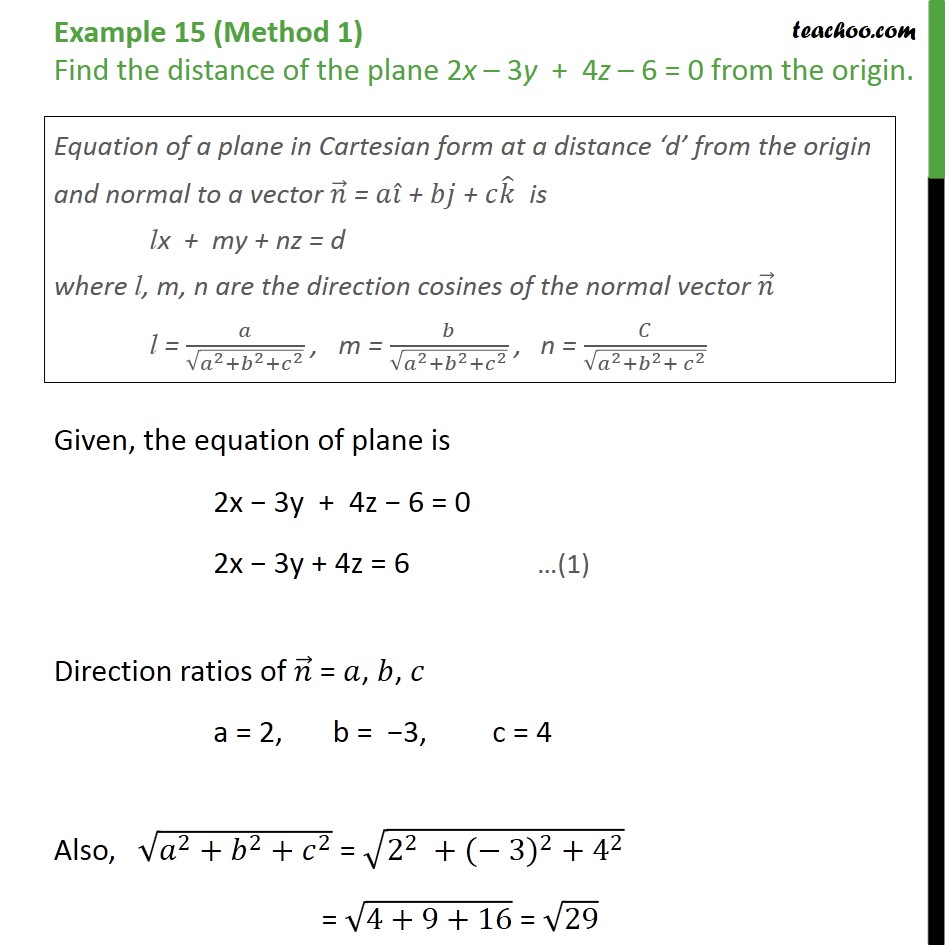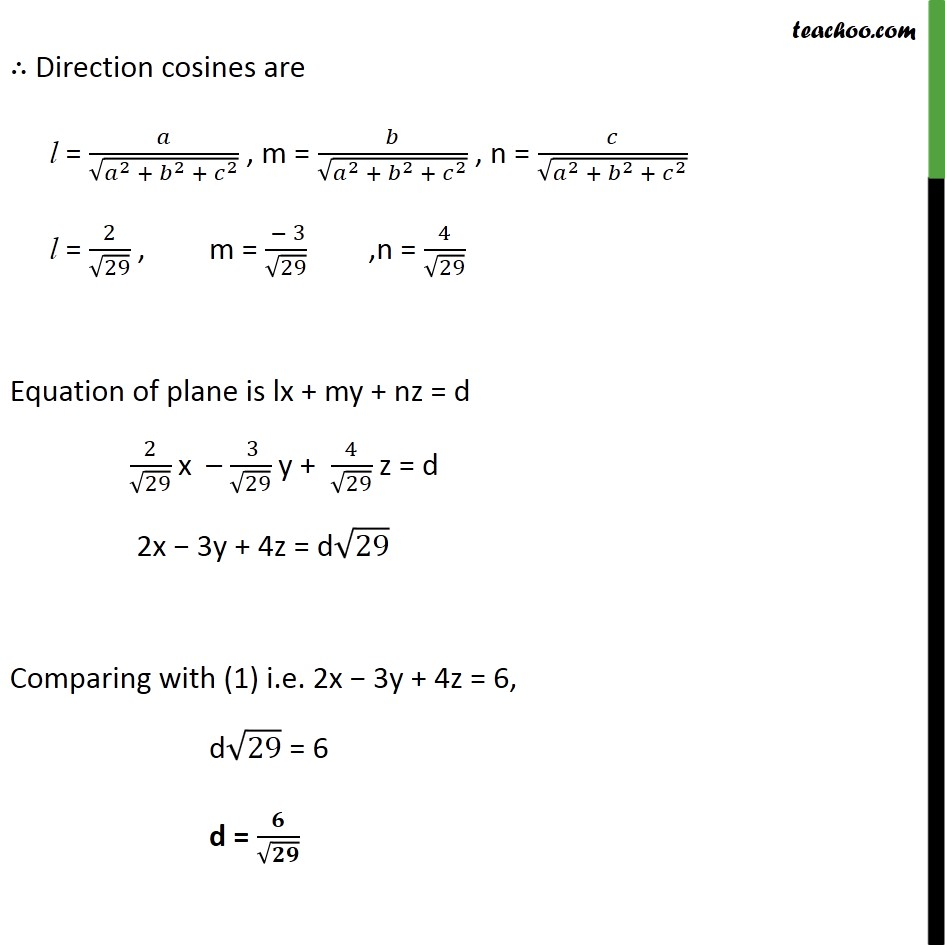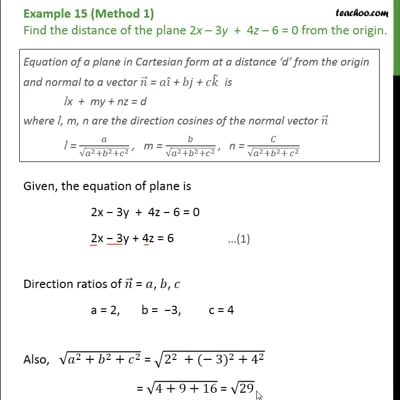This video is only available for Teachoo black users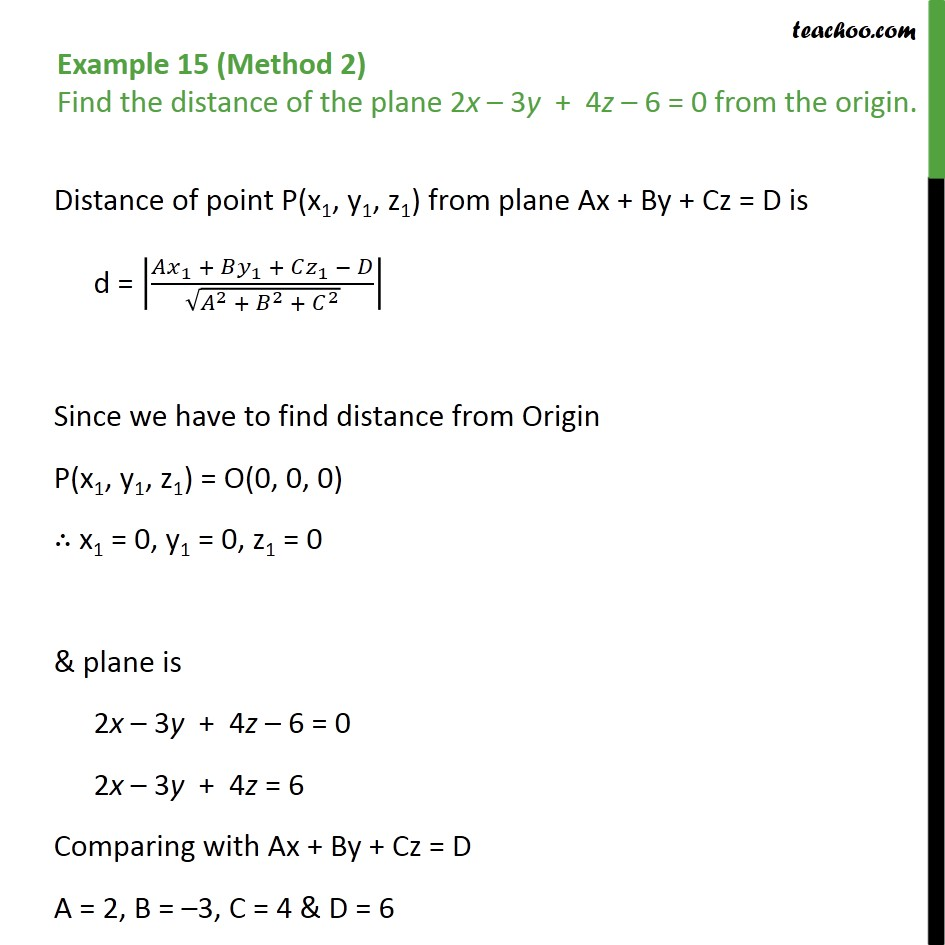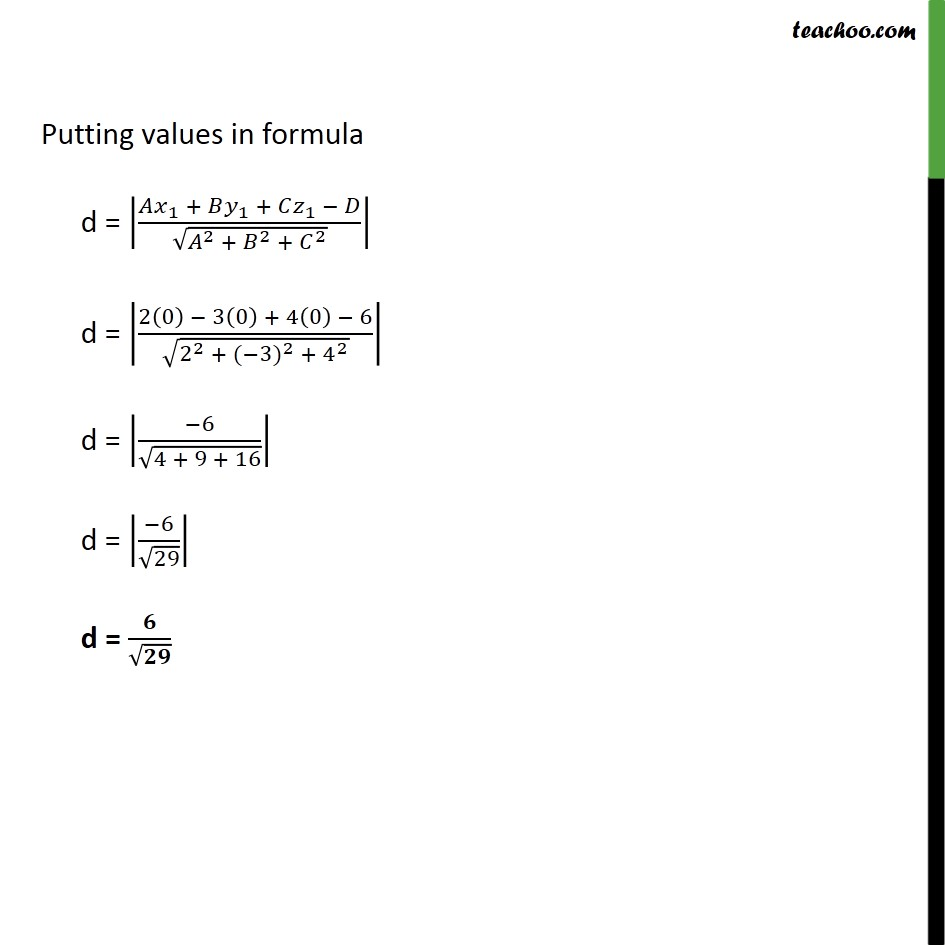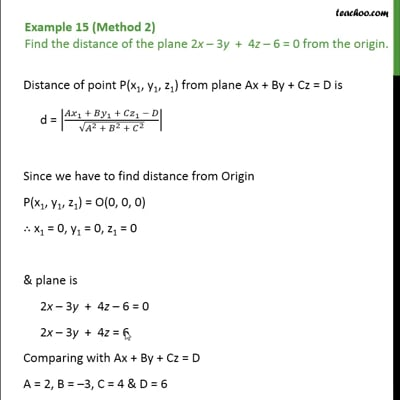This video is only available for Teachoo black users

Introducing your new favourite teacher - Teachoo Black, at only ₹83 per month

### Transcript

Example 15 (Method 1) Find the distance of the plane 2x 3y + 4z 6 = 0 from the origin. Given, the equation of plane is 2x 3y + 4z 6 = 0 2x 3y + 4z = 6 Direction ratios of = , , a = 2, b = 3, c = 4 Also, 2 + 2 + 2 = 2 2 + ( 3) 2 + 4 2 = 4+9+16 = 29 Direction cosines are l = 2 + 2 + 2 , m = 2 + 2 + 2 , n = 2 + 2 + 2 l = 2 29 , m = 3 29 ,n = 4 29 Equation of plane is lx + my + nz = d 2 29 x 3 29 y + 4 29 z = d 2x 3y + 4z = d 29 Comparing with (1) i.e. 2x 3y + 4z = 6, d 29 = 6 d = Example 15 (Method 2) Find the distance of the plane 2x 3y + 4z 6 = 0 from the origin. Distance of point P(x1, y1, z1) from plane Ax + By + Cz = D is d = 1 + 1 + 1 2 + 2 + 2 Since we have to find distance from Origin P(x1, y1, z1) = O(0, 0, 0) x1 = 0, y1 = 0, z1 = 0 & plane is 2x 3y + 4z 6 = 0 2x 3y + 4z = 6 Comparing with Ax + By + Cz = D A = 2, B = 3, C = 4 & D = 6 Putting values in formula d = 1 + 1 + 1 2 + 2 + 2 d = 2 0 3 0 + 4 0 6 2 2 + ( 3) 2 + 4 2 d = 6 4 + 9 + 16 d = 6 29 d =